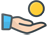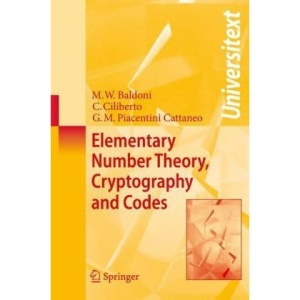30 Day Refund Guarantee# Elementary Number Theory, Cryptography and Codes (Universitext)

by M . Welleda Baldoni (Author), Ciro Ciliberto (Author), Daniele Gewurz (Translator), G . M . Piacentini Cattaneo (Author)

##### Synopsis

In this volume one finds basic techniques from algebra and number theory (e.g. congruences, unique factorization domains, finite fields, quadratic residues, primality tests, continued fractions, etc.) which in recent years have proven to be extremely useful for applications to cryptography and coding theory. Both cryptography and codes have crucial applications in our daily lives, and they are described here, while the complexity problems that arise in implementing the related numerical algorithms are also taken into due account. Cryptography has been developed in great detail, both in its classical and more recent aspects. In particular public key cryptography is extensively discussed, the use of algebraic geometry, specifically of elliptic curves over finite fields, is illustrated, and a final chapter is devoted to quantum cryptography, which is the new frontier of the field. Coding theory is not discussed in full; however a chapter, sufficient for a good introduction to the subject, has been devoted to linear codes. Each chapter ends with several complements and with an extensive list of exercises, the solutions to most of which are included in the last chapter. Though the book contains advanced material, such as cryptography on elliptic curves, Goppa codes using algebraic curves over finite fields, and the recent AKS polynomial primality test, the authors' objective has been to keep the exposition as self-contained and elementary as possible. Therefore the book will be useful to students and researchers, both in theoretical (e.g. mathematicians) and in applied sciences (e.g. physicists, engineers, computer scientists, etc.) seeking a friendly introduction to the important subjects treated here. The book will also be useful for teachers who intend to give courses on these topics.

Quantity

10 in stock